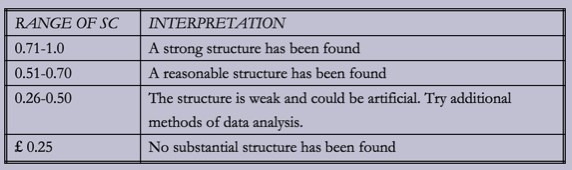# scikit-learn: Clustering and the curse of dimensionality

In my last post I attempted to cluster Game of Thrones episodes based on character appearances without much success. After I wrote that post I was flicking through the scikit-learn clustering documentation and noticed the following section which describes some of the weaknesses of the K-means clustering algorithm:

Inertia is not a normalized metric: we just know that lower values are better and zero is optimal. But in very high-dimensional spaces, Euclidean distances tend to become inflated (this is an instance of the so-called “curse of dimensionality”). Running a dimensionality reduction algorithm such as PCA prior to k-means clustering can alleviate this problem and speed up the computations.

Each episode has 638 dimensions so this is probably the problem we're seeing. I actually thought the 'curse of dimensionality' referred to the greater than linear increase in computation time; I hadn't realised it could also impact the clustering itself.

As the documentation notes, the K-Means algorithm calculates euclidean distances to work out which cluster episodes should go in. Episodes in the same cluster should have a small euclidean distance and items in different clusters should have larger ones.

I created a little script to help me understand the curse of dimensionality. I've got 4 pairs of vectors, of size 4, 6, 100, and 600. Half of the items in the vector match and the other half differ. I calculate the cosine similarity and euclidean distance for each pair of vectors:

``````
from sklearn.metrics.pairwise import cosine_similarity
import numpy as np

def distances(a, b):
return np.linalg.norm(a-b), cosine_similarity([a, b])

def mixed(n_zeros, n_ones):
return np.concatenate((np.repeat(, n_ones), np.repeat(, n_zeros)), axis=0)

def ones(n_ones):
return np.repeat(, n_ones)

print distances(mixed(2, 2), ones(4))
print distances(mixed(3, 3), ones(6))
print distances(mixed(50, 50), ones(100))
print distances(mixed(300, 300), ones(600))

(1.4142135623730951, 0.70710678118654746)
(1.7320508075688772, 0.70710678118654768)
(7.0710678118654755, 0.70710678118654757)
(17.320508075688775, 0.70710678118654746)
``````

The euclidean distance for the 600 item vector is 17x larger than for the one containing 4 items despite having the same similarity score.

Having convinced myself that reducing the dimensionality of the vectors could make a difference I reduced the size of the episodes vectors using the the Truncated SVD algorithm before trying K-means clustering again.

First we reduce the dimensionality of the episodes vectors:

``````
from sklearn.decomposition import TruncatedSVD

n_components = 2
reducer = TruncatedSVD(n_components=n_components)
reducer.fit(all)
new_all = reducer.transform(all)
print("%d: Percentage explained: %s\n" % (n_components, reducer.explained_variance_ratio_.sum()))

2: Percentage explained: 0.124579183633
``````

I'm not sure how much I should be reducing the number of dimensions so I thought 2 would an interesting place to start. I'm not sure exactly what the output of the reducer.explained_variance_ratio_ function means so I need to do some more reading to figure out whether it makes sense to carry on with a dimension of 2.

For now though let's try out the clustering algorithm again and see how it gets on:

``````
from sklearn.cluster import KMeans

for n_clusters in range(2, 10):
km = KMeans(n_clusters=n_clusters, init='k-means++', max_iter=100, n_init=1)
cluster_labels = km.fit_predict(new_all)
silhouette_avg = metrics.silhouette_score(new_all, cluster_labels, sample_size=1000)

print n_clusters, silhouette_avg

2 0.559681096025
3 0.498456585461
4 0.524704352941
5 0.441580592398
6 0.44703058946
7 0.447895331824
8 0.433698007009
9 0.459874485986
``````

This time out silhouette scores are much better. I came across a tutorial from the Guide to Advanced Data Analysis which includes a table explaining how to interpret this score:We have a couple of cluster sizes which fit in the 'reasonable structure' and a few just on the edge of fitting in that category.

I tried varying the number of dimensions and found that 3 worked reasonably well, but after that the silhouette score dropped rapidly. Once we reach 30 dimensions the silhouette score is almost the same as if we hadn't reduced dimensionality at all.

I haven't figured out a good way of visualising the results of my experiments where I vary the dimensions and number of clusters so that's something to work on next. I find it quite difficult to see what's going on by just staring at the raw numbers.

I also need to read up on the SVD algorithm to understand when it is/isn't acceptable to reduce dimensions and how much I should be reducing them by.